# High School Math : Limits

## Example Questions

### Example Question #1 : Limits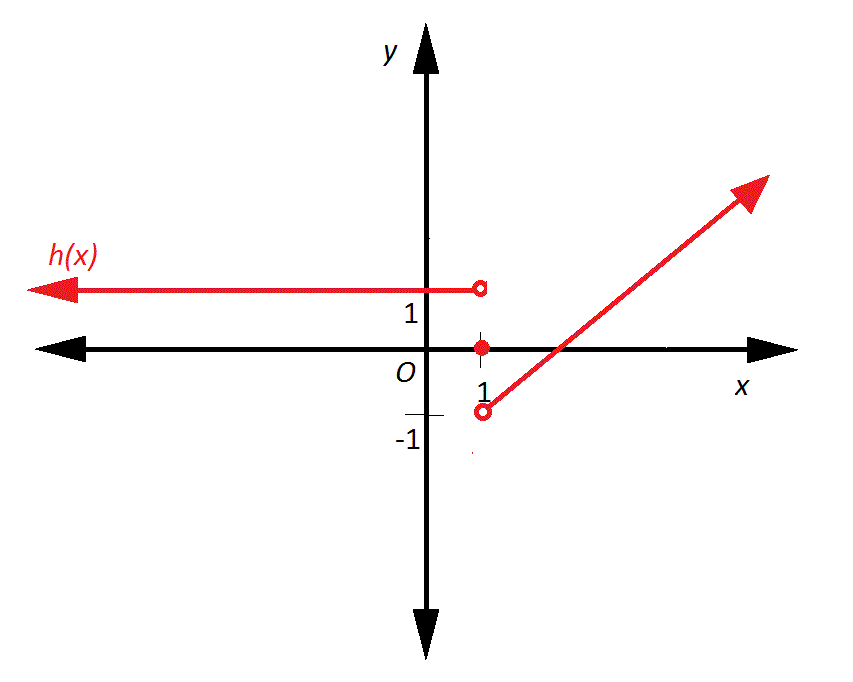The above graph depicts a function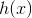. Does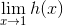exist, and why or why not?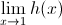does not exist because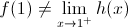does not exist because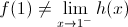exists because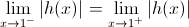does not exist because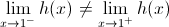.exists because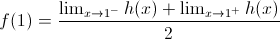does not exist because.

Explanation:exists if and only if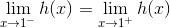. As can be seen from the diagram,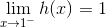, but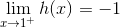. Since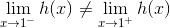,does not exist.

### Example Question #2 : Calculus Ii — Integrals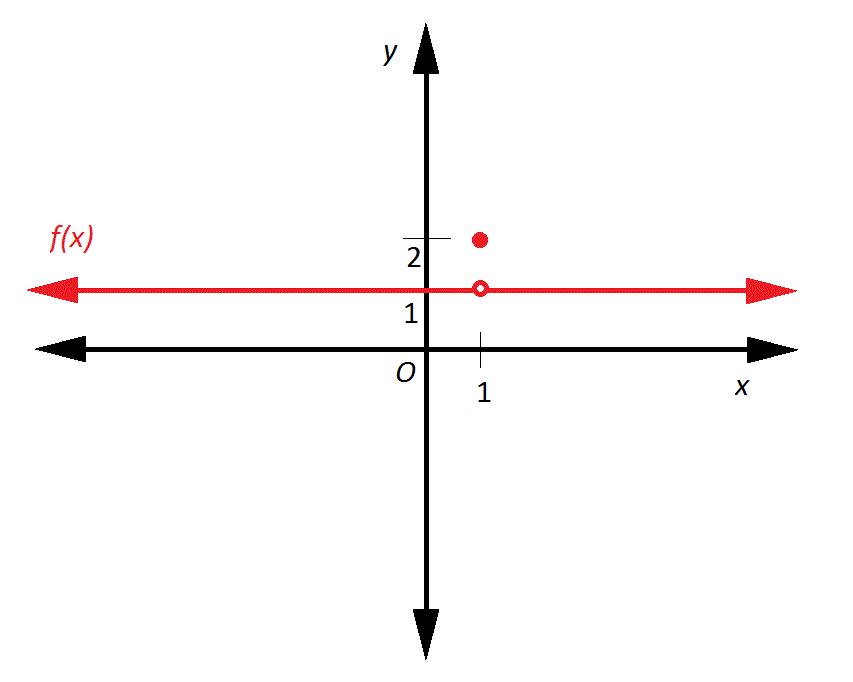The above graph depicts a function. Does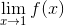exist, and why or why not?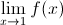does not exist because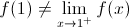exists because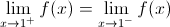does not exist because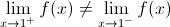does not exist because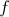is not continuaous at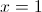.does not exist because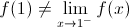exists becauseExplanation:exists if and only if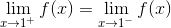;

the actual value ofis irrelevant, as is whetheris continuous there.

As can be seen,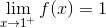and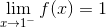;

therefore,,

andexists.

### Example Question #3 : Calculus Ii — Integrals

A function is defined by the following piecewise equation: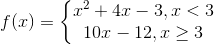At, the function is:

continuous

discontinuous

continuous

Explanation:

The first step to determine continuity at a point is to determine if the function is defined at that point. When we substitute in 3 for, we get 18 as our-value.is thus defined for this function.

The next step is determine if the limit of the function is defined at that point. This means that the left-hand limit must be equal to the right-hand limit at. Substitution reveals the following: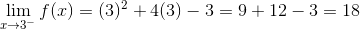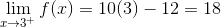Both sides of the function, therefore, approach a-value of 18.

Finally, we must ensure that the curve is smooth by checking the limit of the derivative of both sides.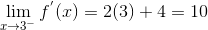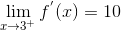Since the function passes all three tests, it is continuous.

### Example Question #4 : Calculus Ii — Integrals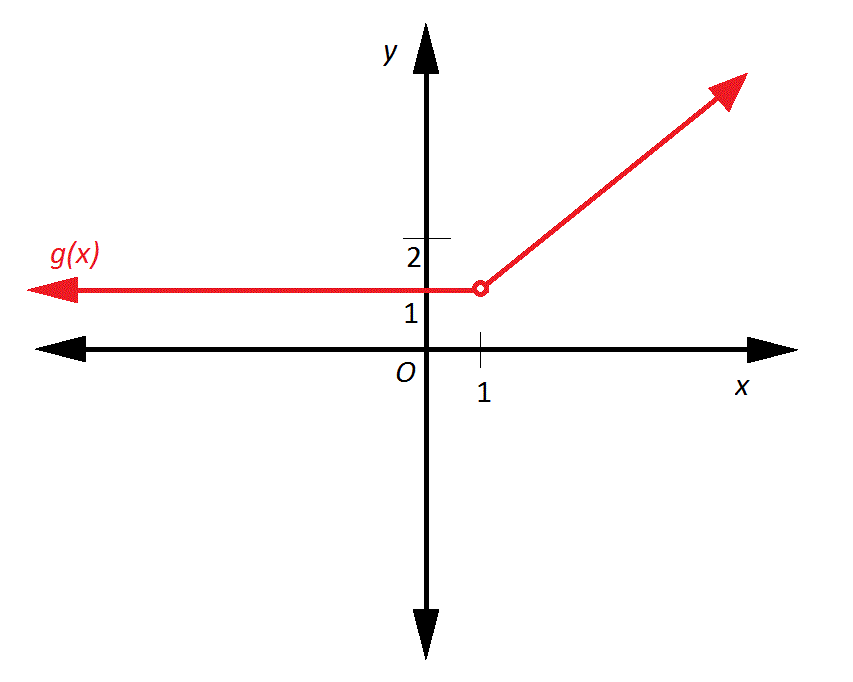The graph depicts a function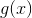. Does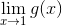exist?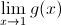does not exist because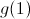is undefined.exists because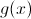is constant on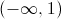.exists because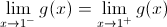.does not exist because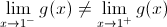.does not exist because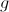is not continuous at.exists because.exists if and only if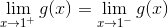; the actual value of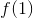is irrelevant.
As can be seen,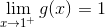and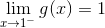; therefore,, andexists.